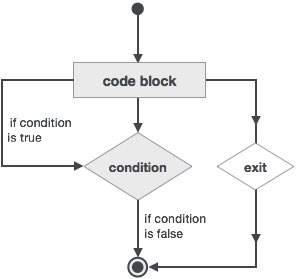# Fortran - Exit Statement

Exit statement terminates the loop or select case statement, and transfers execution to the statement immediately following the loop or select.

## Flow Diagram## Example

```program nestedLoop
implicit none

integer:: i, j, k
iloop: do i = 1, 3
jloop: do j = 1, 3
kloop: do k = 1, 3

print*, "(i, j, k): ", i, j, k

if (k==2) then
exit jloop
end if

end do kloop
end do jloop
end do iloop

end program nestedLoop
```

When the above code is compiled and executed, it produces the following result −

```(i, j, k): 1  1  1
(i, j, k): 1  1  2
(i, j, k): 2  1  1
(i, j, k): 2  1  2
(i, j, k): 3  1  1
(i, j, k): 3  1  2
```
fortran_loops.htm